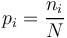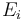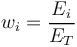# Concentration Index

(Redirected from Concentration Indicator)

## Definition

A concentration index is any Mathematical Expression(function) that converts a distribution of observed values into a single number expressing the prevalence (Concentration) of certain observations amongst the total set.

Concentration indexes find widespread use in (among others) studies of biological diversity, economic inequality, market competition, and Portfolio Management. As the primary means of Concentration Measurement they are indispensable tools in identifying Concentration Risk

## List of General Concentration Indexes

The following list aims to be a complete enumeration of the essentially distinct varieties of concentration indexes:

## Spatial and Geographic Concentration Indexes

When spatial distribution (e.g., geographic location) is an important consideration the above general indexes may have reduced applicability. In this case a specialized type of Geographic Concentration Index might more applicable. Several geographic concentration indexes have been proposed:

• Generalization of the Gini index
• Generalization of the Herfindahl index
• Multigroup Thiel index
• Ellison and Glaeser index
• Maurel and Sedillot index
• Continuous indexes that use distances between locations

## Discrete versus Continuous data sets

There are two possible starting points for input data:

• Observations are discrete, for example$p_i = \frac{n_i}{N}$ is the proportion of a population with total count N that belongs to category i. A number of indexes used in diversity studies (Abundance, Richness, Margalev's, Menhinick’s diversity indexes) are based on species counts.
• Observations are continuous, for example$E_i$ is a range of observations of some continuous quantity E. Such data will typically converted into weights$w_i = \frac{E_i}{E_T}$

## Issues and Challenges

• The very widespread use of concentration indexes means that the same essential functions have been invented many times separately, have been given different names and are used in slightly different manner (inverse relationships, with different normalizations etc.).
• The reduction of Concentration Measurement to a single number is only one of the available quantitative tools to support an analysis. The Lorentz Curve is an example of a more complex approach to studying concentration.